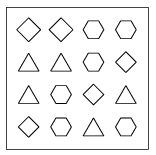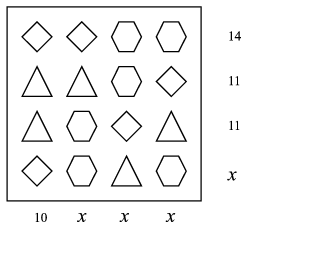Mathematical and Physical Journal
for High Schools
Issued by the MATFUND Foundation
 Already signed up? New to KöMaL?

#Problem K. 99. (November 2006)

K. 99. There are three kind of candies packed in a box, 16 of them altogether. The diagram shows the arrangement of the candies. The total mass of the four ones in the first row is 14 dkg; that of them in the second row is 11 dkg; finally, the total mass of the candies in the first column is 10 dkg. How much is the total mass of the candies in the whole box?(6 pont)

Deadline expired on December 11, 2006.

Sorry, the solution is available only in Hungarian. Google translation

Megoldás. A harmadik sor tömege is 11 dkg. Az ismeretlen negyedik sor, továbbá a második, harmadik és negyedik oszlop tömege azonos.Vagyis 10+3x=14+11+11+x, amiből x=13. A dobozban 49 dkg csokoládé van.

### Statistics:

 258 students sent a solution. 6 points: 187 students. 5 points: 16 students. 4 points: 20 students. 3 points: 13 students. 2 points: 15 students. 1 point: 2 students. 0 point: 3 students. Unfair, not evaluated: 2 solutionss.

Problems in Mathematics of KöMaL, November 2006